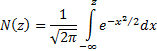/

### Deriving the Black-Scholes Option Pricing Formulae using Ito (stochastic) calculus and partial differential equations

The following partial differential equation is satisfied by the price of any derivative on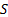, given the assumptions underlying the Black-Scholes world: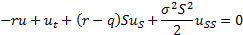This partial differential equation is a second-order, linear partial differential equation of the parabolic type. This type of equation is the same as used by physicists to describe diffusion of heat. For this reason, Gauss-Weiner or Brownian processes are also often commonly called diffusion processes.

If,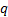and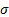are constant then we can solve it by transforming it into a standard form which others have previously solved, namely (for some constant):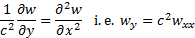This can be achieved by replacing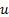by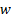where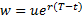(as long asis constant) and by making the following double transformation (assuming that,andare constant):

This transformation removes one of the terms in the partial differential equation: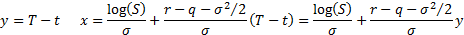The partial differential equation then simplifies to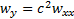, with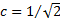. Prices of different derivatives all satisfy this equation and are differentiated by the imposition of different boundary conditions. A common tool for solving partial differential equations subject to such boundary conditions is the use of Green’s functions. This expresses the solution to a partial differential equation given a general boundary condition applicable at some boundary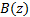, formed say by the curve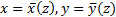, as an expression of the following form, in which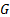is called the Green’s function for that partial differential equation: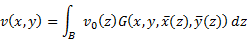The Green’s function forwhereis constant is: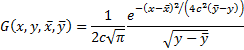If the boundary condition is expressed as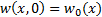at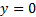, where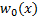is continuous and bounded for all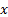, then the solution is: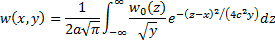For a European call option, with strike price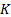, we have, after making the substitutions described above,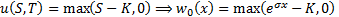. After some further substitutions we find that this implies that: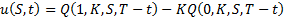where: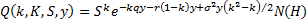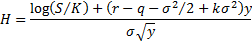Substituting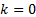and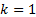into the formula for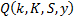recovers the Black-Scholes formulae, e.g. for a call option: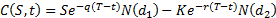Where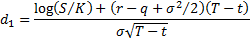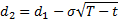and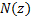is the cumulative Normal distribution function, i.e.